University Physics Volume 1

72.

Two small forces, $F→1=−2.40i^−6.10j^F→1=−2.40i^−6.10j^$ N and $F→2=8.50i^−9.70j^F→2=8.50i^−9.70j^$ N, are exerted on a rogue asteroid by a pair of space tractors. (a) Find the net force. (b) What are the magnitude and direction of the net force? (c) If the mass of the asteroid is 125 kg, what acceleration does it experience (in vector form)? (d) What are the magnitude and direction of the acceleration?

73.

Two forces of 25 and 45 N act on an object. Their directions differ by $70°70°$. The resulting acceleration has magnitude of $10.0m/s2.10.0m/s2.$ What is the mass of the body?

74.

A force of 1600 N acts parallel to a ramp to push a 300-kg piano into a moving van. The ramp is inclined at $20°20°$. (a) What is the acceleration of the piano up the ramp? (b) What is the velocity of the piano when it reaches the top if the ramp is 4.0 m long and the piano starts from rest?

75.

Draw a free-body diagram of a diver who has entered the water, moved downward, and is acted on by an upward force due to the water which balances the weight (that is, the diver is suspended).

76.

For a swimmer who has just jumped off a diving board, assume air resistance is negligible. The swimmer has a mass of 80.0 kg and jumps off a board 10.0 m above the water. Three seconds after entering the water, her downward motion is stopped. What average upward force did the water exert on her?

77.

(a) Find an equation to determine the magnitude of the net force required to stop a car of mass m, given that the initial speed of the car is $v0v0$ and the stopping distance is x. (b) Find the magnitude of the net force if the mass of the car is 1050 kg, the initial speed is 40.0 km/h, and the stopping distance is 25.0 m.

78.

A sailboat has a mass of $1.50×1031.50×103$ kg and is acted on by a force of $2.00×1032.00×103$ N toward the east, while the wind acts behind the sails with a force of $3.00×1033.00×103$ N in a direction $45°45°$ north of east. Find the magnitude and direction of the resulting acceleration.

79.

Find the acceleration of the body of mass 10.0 kg shown below.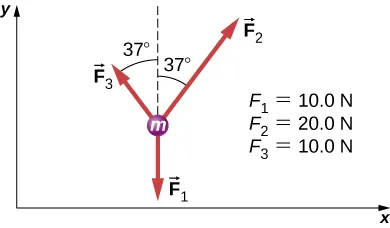80.

A body of mass 2.0 kg is moving along the x-axis with a speed of 3.0 m/s at the instant represented below. (a) What is the acceleration of the body? (b) What is the body’s velocity 10.0 s later? (c) What is its displacement after 10.0 s?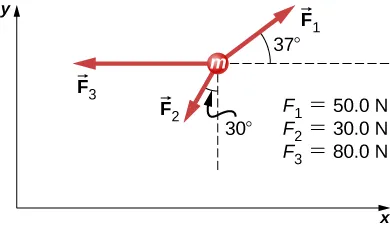81.

Force $F→BF→B$ has twice the magnitude of force $F→A.F→A.$ Find the direction in which the particle accelerates in this figure.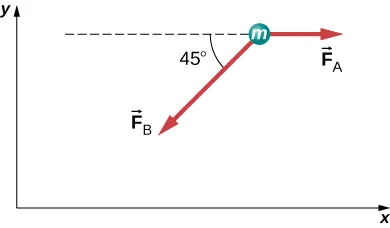82.

Shown below is a body of mass 1.0 kg under the influence of the forces $F→AF→A$, $F→BF→B$, and $mg→mg→$. If the body accelerates to the left at $20m/s220m/s2$, what are $F→AF→A$ and $F→BF→B$?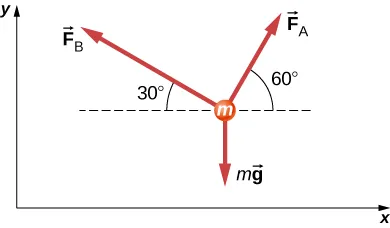83.

A force acts on a car of mass m so that the speed v of the car increases with position x as $v=kx2v=kx2$, where k is constant and all quantities are in SI units. Find the force acting on the car as a function of position.

84.

A 7.0-N force parallel to an incline is applied to a 1.0-kg crate. The ramp is tilted at $20°20°$ and is frictionless. (a) What is the acceleration of the crate? (b) If all other conditions are the same but the ramp has a friction force of 1.9 N, what is the acceleration?

85.

Two boxes, A and B, are at rest. Box A is on level ground, while box B rests on an inclined plane tilted at angle $θθ$ with the horizontal. (a) Write expressions for the normal force acting on each block. (b) Compare the two forces; that is, tell which one is larger or whether they are equal in magnitude. (c) If the angle of incline is $10°10°$, which force is greater?

86.

A mass of 250.0 g is suspended from a spring hanging vertically. The spring stretches 6.00 cm. How much will the spring stretch if the suspended mass is 530.0 g?

87.

As shown below, two identical springs, each with the spring constant 20 N/m, support a 15.0-N weight. (a) What is the tension in spring A? (b) What is the amount of stretch of spring A from the rest position?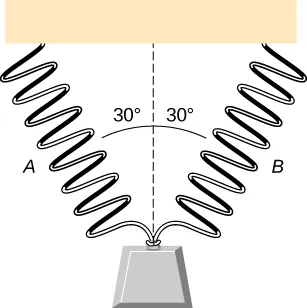88.

Shown below is a 30.0-kg block resting on a frictionless ramp inclined at $60°60°$ to the horizontal. The block is held by a spring that is stretched 5.0 cm. What is the force constant of the spring?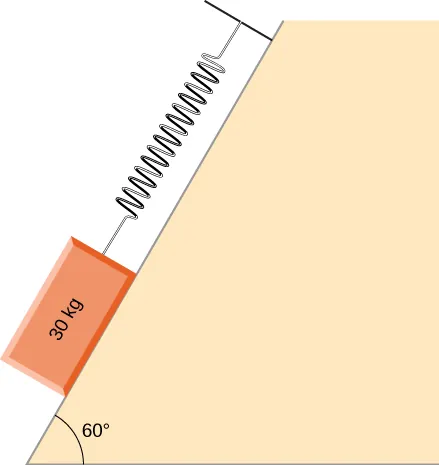89.

In building a house, carpenters use nails from a large box. The box is suspended from a spring twice during the day to measure the usage of nails. At the beginning of the day, the spring stretches 50 cm. At the end of the day, the spring stretches 30 cm. What fraction or percentage of the nails have been used?

90.

A force is applied to a block to move it up a $30°30°$ incline. The incline is frictionless. If $F=65.0NF=65.0N$ and $M=5.00kgM=5.00kg$, what is the magnitude of the acceleration of the block?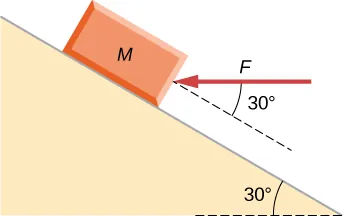91.

Two forces are applied to a 5.0-kg object, and it accelerates at a rate of $2.0m/s22.0m/s2$ in the positive y-direction. If one of the forces acts in the positive x-direction with magnitude 12.0 N, find the magnitude of the other force.

92.

The block on the right shown below has more mass than the block on the left ($m2>m1m2>m1$). Draw free-body diagrams for each block.Order a print copy

As an Amazon Associate we earn from qualifying purchases.